How Cheenta works to ensure student success?
Explore the Back-Story
Get inspired by the success stories of our students in IIT JAM MS, ISI  MStat, CMI MSc DS.  Learn More

# 2016 ISI Objective Solution Problem 1## Understand the Problem:

The polynomial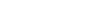is divisible by

• (A)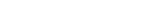• (B)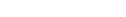• (C)• (D)## Solution

The problem is easy to understand. Your first task is to try the problem yourself.

Try to factorize.

Observe that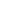and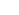are the roots of.=  (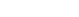).()

A shorter solution or approach can always exist. Think about it. If you find an alternative solution or approach, mention it in the comments. We would love to hear something different from you.

Also Visit: I.S.I. & C.M.I Entrance Program

## Understand the Problem:

The polynomialis divisible by

• (A)• (B)• (C)• (D)## Solution

The problem is easy to understand. Your first task is to try the problem yourself.

Try to factorize.

Observe thatandare the roots of.=  ().()

A shorter solution or approach can always exist. Think about it. If you find an alternative solution or approach, mention it in the comments. We would love to hear something different from you.

Also Visit: I.S.I. & C.M.I Entrance Program

This site uses Akismet to reduce spam. Learn how your comment data is processed.

### 5 comments on “2016 ISI Objective Solution Problem 1”

1.DEB JYOTI MITRA says:

f(x)=(x^7+x^2+1);
g(x)=(x^2+x+1);
we observe
f(x)/g(x)=(x^5-x^4+x^2-x+1) using WolframAlpha

1.Srijit Mukherjee says:

Yes, you are right!:)

2.DEB MITRA says:

thanks

3.Ramesh Knowledge Index says:

I did it using the theory of equation.
Sum of roots of the given polynomial x^7 + x^2 + 1 = 0
if Option one is true then it must be multiplied to x^2 + x + 1 as the sum of roots of the polynomial in option one is 1. Multiplying x^5 - x^4 + x^2 -x + 1 with x^2+x+1 yields us the required polynomial.
I was lucky that the first option itself was correct. But even if it wouid not have been then finding the pair and multilying would not have been that long as the given polynomials are easy to multiply

4.Bangalore Mathematical Institute says:

By 1+w+w^2=0 , where 'w' is comlex cube root of unity. we see that 'w' and 'w^2' satisfy x^7+x^2+1=0. So x^2+x+1 is a factor.

### Knowledge Partner